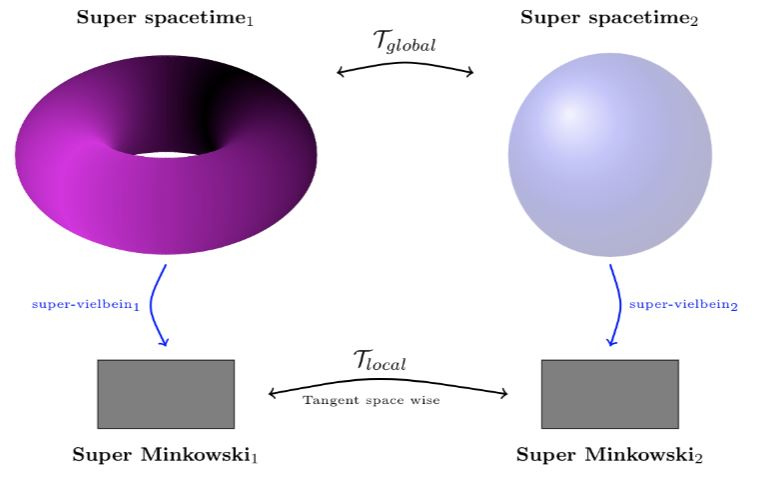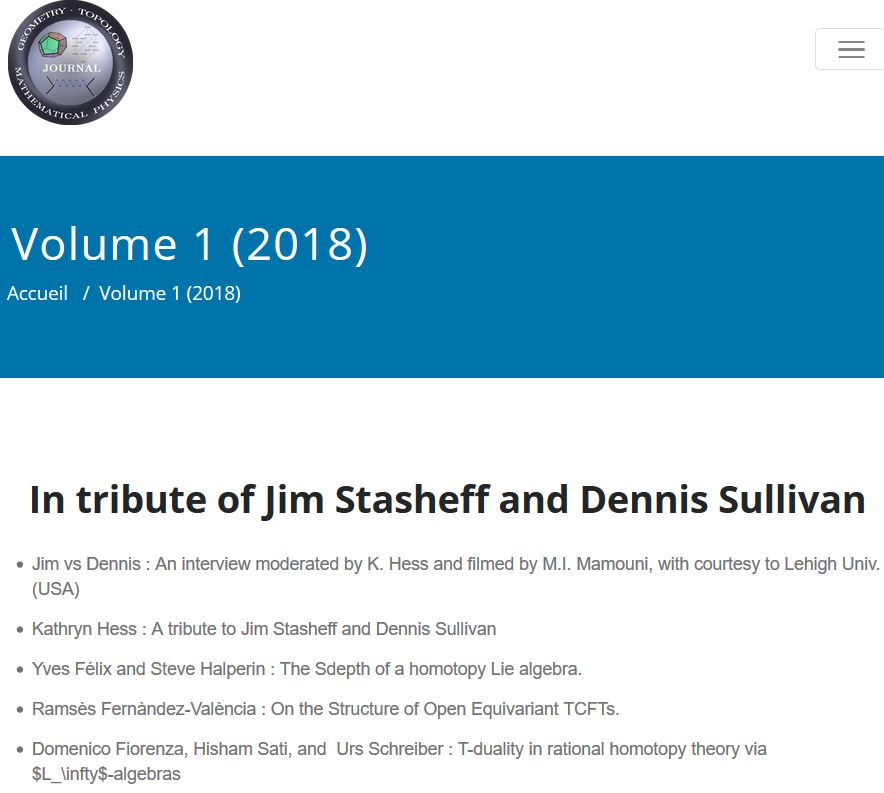# Schreiber T-Duality from super Lie n-algebra cocycles for super p-branes

An article that we have written:Abstract. We compute the L-infinity-theoretic double dimensional reduction of the F1/D$p$-brane super $L_\infty$-cocycles with coefficients in rationalized twisted K-theory from the 10d type IIA and type IIB super Lie algebras down to 9d. We show that the two resulting coefficient $L_\infty$-algebras are naturally related by an $L_\infty$-isomorphism which we find to act on the super $p$-brane cocycles by the infinitesimal version of the rules of topological T-duality and inducing an isomorphism between $K^0$ and $K^1$, rationally. Moreover, we show that these $L_\infty$-algebras are the homotopy quotients of the RR-charge coefficients by the “T-duality Lie 2-algebra”. We find that the induced $L_\infty$-extension is a gerby extension of a $9+(1,1)$ dimensional (i.e. “doubled”) T-duality correspondence super-spacetime, which serves as a local model for T-folds. We observe that this still extends, via the D0-brane cocycle of its type IIA factor, to a $10+(1,1)$-dimensional super Lie algebra. Finally we show that this satisfies all the expected properties of a local model space for F-theory elliptic fibrations.

$\,$

Talk slides:Exposition

of the underlying rational topological T-duality:

Lecture notes:

Previous articles:

Further developments

summed up in

$\,$

Last revised on October 5, 2019 at 07:11:01. See the history of this page for a list of all contributions to it.